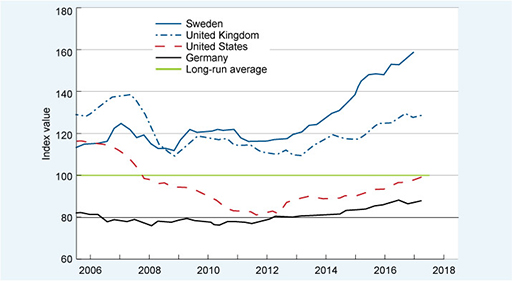### Become an OU studentStart this free course now. Just create an account and sign in. Enrol and complete the course for a free statement of participation or digital badge if available.

# 2.6 The house price-to-income ratio

The house price-to-income ratio takes the basic form of:

• Average house price ÷ Average income of buyers

This ratio is the starting point for the data shown in Figure 6. However, some adjustments have been made so that, for each country, the ratio in any period can be compared with its long-run average value. First, the average of the ratio has been calculated over a long period (several decades). The ratio for each period has then been divided by this long-run average. Finally, the answers have then been turned into index values, and it is those index values that are plotted in the chart in Figure 6. You looked at index values earlier – recall that they show the value of something (in this case how the house-price-to-income ratio compares with its long-run average) relative to a base value which is set at 100.

These adjustments may seem complicated but they mean we can describe house prices relative to income as being high or low relative to the long-run value of the ratio. And the adjustments strip out the differences caused by different currencies and different average house price levels between countries. If the figure for a particular period:

• equals 100, this means the house price-to-income ratio is the same as the long-run average
• is lower than 100, the ratio is below the long-run average and might suggest home buying is relatively cheap
• is higher than 100, the ratio is above the long-run average and this might suggest that house prices are high – or even too high – compared to average incomes.Figure 6 House price-to-income ratios relative to their long-run average for selected countries, 2006–2017. Source: authors’ chart using data from OECD (2018). Index: base value is the long-run average = 100.